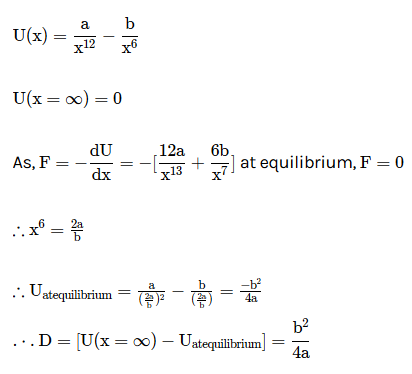# The potential energy of a diatomic molecule is given by U = A/r12 - B/r6 where r is the distance between the atoms that make up the molecule and A,B are positive constants. Find the equilibrium separation betweeen atoms

10 years ago

for equilibrium positions  dU/dR= -F must be zero

U=A/r12-B/r6

dU/dR=  -12A/r13+6B/r7=0

B/r7=2A/r13

r6=2A/B

r=(2A/B)1/6

2 years ago
Dear Student,